图像处理 测地距离_图像测距离 - CSDN
• 学习基于测地距离图像分割算法，是由于之前把grab cut算法实现了一遍，感觉效果很坑爹，于是就尝试基于测地距离的分割算法。这个算法开始前，需要先非常熟悉“测地距离”这个概念，因为之前写过三角网格曲

这段时间为了搞项目，涉及到图像分割算法，由于感觉传统的分割算法得出来的效果都很差。于是就尝试各种图像分割算法，把每种分割算法的代码都写一写，一来是为了提高自己的编程能力，二来是为了更加深刻的了解算法。学习基于测地距离的图像分割算法，是由于之前把grab cut算法实现了一遍，感觉效果很坑爹，于是就尝试基于测地距离的分割算法。这个算法开始前，需要先非常熟悉“测地距离”这个概念，因为之前写过三角网格曲面上的Dijkstra算法，在三维曲面空间中两点间的测地距离就是两点间沿着曲面走的最短路径，因为之前对测地距离这个概念就比较熟悉，所以看《Geodesic Matting： A Framework for Fast Interactive Image》这篇paper，也就觉得没有什么难度，所以果断写代码，试验一下效果再说。

1、算法原理：基于测地距离的图像分割属于一种图论的分割算法。图论分割算法：即把图像上的每个像素点当做图的顶点，图的每个顶点有四个邻接顶点（每个像素点有四个邻接像素点，除边界点），每两个邻接像素点用相应的边连接，边的长度与两个像素点间的相似度有关（测地距离），而非采用简单的欧式距离作为边长（相邻像素点间的欧式距离为1），具体文献中边长的计算方法见下文。

2、基于测地距离的分割算法流程：给定一张待分割的图片，首先算法会利用该图片构造一张距离图，边的距离大小：参考文献中采用的是现根据已知的标记点前景、背景，对未知的像素点进行核密度估计，并根据相邻相似点间的隶属于前景的概率差，作为边长，我写的demo中是直接采用像素值的差值作为边长。

具体实现:在测地距离的算法中提供两种类型的笔刷，其作用是用来标记为分割时的前景F和相应的背景B。

①首先会需要用户在相应的前景和背景区域做标记。

②计算未标记点到已标记点前景的测地距离d1，及背景的测地距离d2。测地距离的计算方法我的demo中采用的是Dijkstra算法进行计算(Dijkstra算法原理请百度搜索)。具体过程如下：首先，把标号为的标记点到目标的测地距离初始化为0，到背景的测地距离初始化为无穷大；同样，把标号为的标记点到背景的测地距离初始化为0，到目标的测地距离初始化为无穷大。根据连通标号矩阵，搜索标号为的所有标记点的4连通邻域像素点，找出这些像素点中权重概率最小的那个像素点，把最小权重概率对应的像素点添加到已排好序的路径上，并通过比较原来的权重概率与加入新顶点后的新概率大小，决定是否更新其它像素点的权重概率。这样，不断地添加新的像素点到已排好序的路径上，直到图像中所有像素点全部搜索完为止。于是由一个或多个权重概率加和得到每个像素点到目标的测地距离d1(x)。同理，可以计算出图像中各像素点到背景的测地距离d2(x)。

③根据测地距离的大小，判断未标记点属于前景或背景。根据概率公式，由图像中的每个像素点到目标和背景的测地距离，计算出该像素点分属于目标和背景的概率：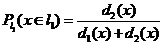，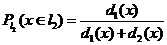对于图像中的各像素点，若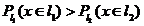，则把该像素点判为目标像素点则判为把该像素点判为背景像素点。

看一下我测试的几张图片分割效果：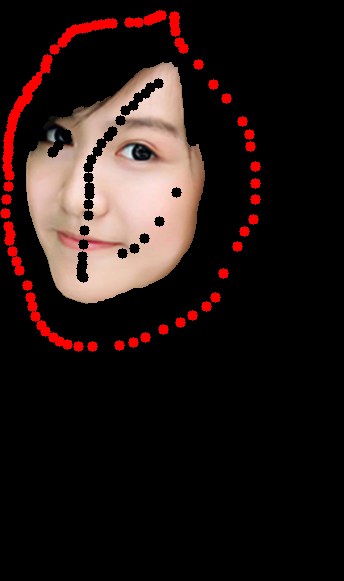原图                                                                   分割结果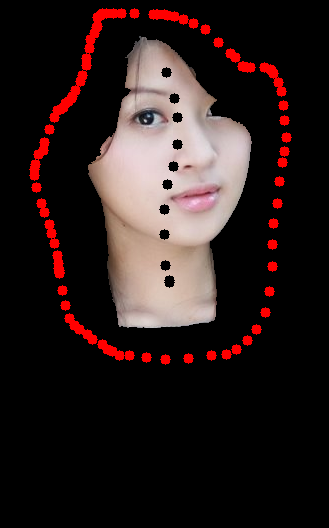原图                                                                                         分割结果

最后总结一下算法的优缺点：这个算法给我的感觉，至少比grab cut 算法强，2004年的grab  cut 算法paper效果看起看挺不错的，但是试验起来感觉很坑爹。

算法优点：计算速度快，比grab cut算法快好多(demo未做整理，边长重复计算，因此速度达不到文献中速度)，对图片中包含半透明区域的图像分割也有一定的效果。

算法缺点：如果前景和背景边缘比较模糊，这时测地距离的图像分割容易出错。这时候用户如果想得到一个较好的分割效果，需要额外的交互或者对图像进行其他的处理。

附：还有一篇2010年文献《Geodesic graph cut for interactive image segmentation》结合了graph cut 算法和测地距离分割算法的优点，把两种算法结合起来，可克服两种算法存在的缺陷。

总结：图像分割算法基本上鲁棒性都很差，加点噪声，边缘较弱等对所有的图像分割算法影响都很大，算法一般只对文献上的图片效果较好。

本文地址：http://blog.csdn.net/hjimce/article/details/45221607
作者：hjimce     联系qq：1393852684

更多资源请关注我的博客：http://blog.csdn.net/hjimce
原创文章，转载请保留这两行作者信息

展开全文图像处理 图像分割 测地距离
• 图像处理之霍夫变换（直线检测算法） 霍夫变换是图像变换中的经典手段之一，主要用来从图像中分离出具有某种相同特征的几何 形状（如，直线，圆等）。霍夫变换寻找直线与圆的方法相比与其它方法可以更好的减少噪 声...
图像处理之霍夫变换（直线检测算法）霍夫变换是图像变换中的经典手段之一，主要用来从图像中分离出具有某种相同特征的几何形状（如，直线，圆等）。霍夫变换寻找直线与圆的方法相比与其它方法可以更好的减少噪声干扰。经典的霍夫变换常用来检测直线，圆，椭圆等。 霍夫变换算法思想：以直线检测为例，每个像素坐标点经过变换都变成都直线特质有贡献的统一度量，一个简单的例子如下：一条直线在图像中是一系列离散点的集合，通过一个直线的离散极坐标公式，可以表达出直线的离散点几何等式如下：X *cos(theta) + y * sin(theta)  = r 其中角度theta指r与X轴之间的夹角，r为到直线几何垂直距离。任何在直线上点，x, y都可以表达，其中 r， theta是常量。该公式图形表示如下：然而在实现的图像处理领域，图像的像素坐标P(x, y)是已知的，而r, theta则是我们要寻找的变量。如果我们能绘制每个(r, theta)值根据像素点坐标P(x, y)值的话，那么就从图像笛卡尔坐标系统转换到极坐标霍夫空间系统，这种从点到曲线的变换称为直线的霍夫变换。变换通过量化霍夫参数空间为有限个值间隔等分或者累加格子。当霍夫变换算法开始，每个像素坐标点P(x, y)被转换到(r, theta)的曲线点上面，累加到对应的格子数据点，当一个波峰出现时候，说明有直线存在。同样的原理，我们可以用来检测圆，只是对于圆的参数方程变为如下等式：(x –a ) ^2 + (y-b) ^ 2 = r^2其中(a, b)为圆的中心点坐标，r圆的半径。这样霍夫的参数空间就变成一个三维参数空间。给定圆半径转为二维霍夫参数空间，变换相对简单，也比较常用。 编程思路解析：1.      读取一幅带处理二值图像，最好背景为黑色。2.      取得源像素数据3.      根据直线的霍夫变换公式完成霍夫变换，预览霍夫空间结果4.       寻找最大霍夫值，设置阈值，反变换到图像RGB值空间(程序难点之一)5.      越界处理，显示霍夫变换处理以后的图像 关键代码解析：直线的变换角度为[0 ~ PI]之间，设置等份为500为PI/500，同时根据参数直线参数方程的取值范围为[-r, r]有如下霍夫参数定义： // prepare for hough transform
int centerX = width / 2;
int centerY = height / 2;
double hough_interval = PI_VALUE/(double)hough_space;

int max = Math.max(width, height);
int max_length = (int)(Math.sqrt(2.0D) * max);
hough_1d = new int[2 * hough_space * max_length];实现从像素RGB空间到霍夫空间变换的代码为：// start hough transform now....
int[][] image_2d = convert1Dto2D(inPixels);
for (int row = 0; row < height; row++) {
for (int col = 0; col < width; col++) {
int p = image_2d[row][col] & 0xff;
if(p == 0) continue; // which means background color

// since we does not know the theta angle and r value,
// we have to calculate all hough space for each pixel point
// then we got the max possible theta and r pair.
// r = x * cos(theta) + y * sin(theta)
for(int cell=0; cell < hough_space; cell++ ) {
max = (int)((col - centerX) * Math.cos(cell * hough_interval) + (row - centerY) * Math.sin(cell * hough_interval));
max += max_length; // start from zero, not (-max_length)
if (max < 0 || (max >= 2 * max_length)) {// make sure r did not out of scope[0, 2*max_lenght]
continue;
}
hough_2d[cell][max] +=1;
}
}
}寻找最大霍夫值计算霍夫阈值的代码如下：// find the max hough value
int max_hough = 0;
for(int i=0; i<hough_space; i++) {
for(int j=0; j<2*max_length; j++) {
hough_1d[(i + j * hough_space)] = hough_2d[i][j];
if(hough_2d[i][j] > max_hough) {
max_hough = hough_2d[i][j];
}
}
}
System.out.println("MAX HOUGH VALUE = " + max_hough);

// transfer back to image pixels space from hough parameter space
int hough_threshold = (int)(threshold * max_hough);从霍夫空间反变换回像素数据空间代码如下：	    // transfer back to image pixels space from hough parameter space
int hough_threshold = (int)(threshold * max_hough);
for(int row = 0; row < hough_space; row++) {
for(int col = 0; col < 2*max_length; col++) {
if(hough_2d[row][col] < hough_threshold) // discard it
continue;
int hough_value = hough_2d[row][col];
boolean isLine = true;
for(int i=-1; i<2; i++) {
for(int j=-1; j<2; j++) {
if(i != 0 || j != 0) {
int yf = row + i;
int xf = col + j;
if(xf < 0) continue;
if(xf < 2*max_length) {
if (yf < 0) {
yf += hough_space;
}
if (yf >= hough_space) {
yf -= hough_space;
}
if(hough_2d[yf][xf] <= hough_value) {
continue;
}
isLine = false;
break;
}
}
}
}
if(!isLine) continue;

// transform back to pixel data now...
double dy = Math.sin(row * hough_interval);
double dx = Math.cos(row * hough_interval);
if ((row <= hough_space / 4) || (row >= 3 * hough_space / 4)) {
for (int subrow = 0; subrow < height; ++subrow) {
int subcol = (int)((col - max_length - ((subrow - centerY) * dy)) / dx) + centerX;
if ((subcol < width) && (subcol >= 0)) {
image_2d[subrow][subcol] = -16776961;
}
}
} else {
for (int subcol = 0; subcol < width; ++subcol) {
int subrow = (int)((col - max_length - ((subcol - centerX) * dx)) / dy) + centerY;
if ((subrow < height) && (subrow >= 0)) {
image_2d[subrow][subcol] = -16776961;
}
}
}
}
}霍夫变换源图如下：霍夫变换以后，在霍夫空间显示如下：（白色表示已经找到直线信号）最终反变换回到像素空间效果如下：一个更好的运行监测直线的结果(输入为二值图像)：完整的霍夫变换源代码如下：package com.gloomyfish.image.transform;

import java.awt.image.BufferedImage;

import com.process.blur.study.AbstractBufferedImageOp;

public class HoughLineFilter extends AbstractBufferedImageOp {
public final static double PI_VALUE = Math.PI;
private int hough_space = 500;
private int[] hough_1d;
private int[][] hough_2d;
private int width;
private int height;

private float threshold;
private float scale;
private float offset;

public HoughLineFilter() {
// default hough transform parameters
//	scale = 1.0f;
//	offset = 0.0f;
threshold = 0.5f;
scale = 1.0f;
offset = 0.0f;
}

public void setHoughSpace(int space) {
this.hough_space = space;
}

public float getThreshold() {
return threshold;
}

public void setThreshold(float threshold) {
this.threshold = threshold;
}

public float getScale() {
return scale;
}

public void setScale(float scale) {
this.scale = scale;
}

public float getOffset() {
return offset;
}

public void setOffset(float offset) {
this.offset = offset;
}

@Override
public BufferedImage filter(BufferedImage src, BufferedImage dest) {
width = src.getWidth();
height = src.getHeight();

if ( dest == null )
dest = createCompatibleDestImage( src, null );

int[] inPixels = new int[width*height];
int[] outPixels = new int[width*height];
getRGB( src, 0, 0, width, height, inPixels );
houghTransform(inPixels, outPixels);
setRGB( dest, 0, 0, width, height, outPixels );
return dest;
}

private void houghTransform(int[] inPixels, int[] outPixels) {
// prepare for hough transform
int centerX = width / 2;
int centerY = height / 2;
double hough_interval = PI_VALUE/(double)hough_space;

int max = Math.max(width, height);
int max_length = (int)(Math.sqrt(2.0D) * max);
hough_1d = new int[2 * hough_space * max_length];

// define temp hough 2D array and initialize the hough 2D
hough_2d = new int[hough_space][2*max_length];
for(int i=0; i<hough_space; i++) {
for(int j=0; j<2*max_length; j++) {
hough_2d[i][j] = 0;
}
}

// start hough transform now....
int[][] image_2d = convert1Dto2D(inPixels);
for (int row = 0; row < height; row++) {
for (int col = 0; col < width; col++) {
int p = image_2d[row][col] & 0xff;
if(p == 0) continue; // which means background color

// since we does not know the theta angle and r value,
// we have to calculate all hough space for each pixel point
// then we got the max possible theta and r pair.
// r = x * cos(theta) + y * sin(theta)
for(int cell=0; cell < hough_space; cell++ ) {
max = (int)((col - centerX) * Math.cos(cell * hough_interval) + (row - centerY) * Math.sin(cell * hough_interval));
max += max_length; // start from zero, not (-max_length)
if (max < 0 || (max >= 2 * max_length)) {// make sure r did not out of scope[0, 2*max_lenght]
continue;
}
hough_2d[cell][max] +=1;
}
}
}

// find the max hough value
int max_hough = 0;
for(int i=0; i<hough_space; i++) {
for(int j=0; j<2*max_length; j++) {
hough_1d[(i + j * hough_space)] = hough_2d[i][j];
if(hough_2d[i][j] > max_hough) {
max_hough = hough_2d[i][j];
}
}
}
System.out.println("MAX HOUGH VALUE = " + max_hough);

// transfer back to image pixels space from hough parameter space
int hough_threshold = (int)(threshold * max_hough);
for(int row = 0; row < hough_space; row++) {
for(int col = 0; col < 2*max_length; col++) {
if(hough_2d[row][col] < hough_threshold) // discard it
continue;
int hough_value = hough_2d[row][col];
boolean isLine = true;
for(int i=-1; i<2; i++) {
for(int j=-1; j<2; j++) {
if(i != 0 || j != 0) {
int yf = row + i;
int xf = col + j;
if(xf < 0) continue;
if(xf < 2*max_length) {
if (yf < 0) {
yf += hough_space;
}
if (yf >= hough_space) {
yf -= hough_space;
}
if(hough_2d[yf][xf] <= hough_value) {
continue;
}
isLine = false;
break;
}
}
}
}
if(!isLine) continue;

// transform back to pixel data now...
double dy = Math.sin(row * hough_interval);
double dx = Math.cos(row * hough_interval);
if ((row <= hough_space / 4) || (row >= 3 * hough_space / 4)) {
for (int subrow = 0; subrow < height; ++subrow) {
int subcol = (int)((col - max_length - ((subrow - centerY) * dy)) / dx) + centerX;
if ((subcol < width) && (subcol >= 0)) {
image_2d[subrow][subcol] = -16776961;
}
}
} else {
for (int subcol = 0; subcol < width; ++subcol) {
int subrow = (int)((col - max_length - ((subcol - centerX) * dx)) / dy) + centerY;
if ((subrow < height) && (subrow >= 0)) {
image_2d[subrow][subcol] = -16776961;
}
}
}
}
}

// convert to hough 1D and return result
for (int i = 0; i < this.hough_1d.length; i++)
{
int value = clamp((int)(scale * this.hough_1d[i] + offset)); // scale always equals 1
this.hough_1d[i] = (0xFF000000 | value + (value << 16) + (value << 8));
}

// convert to image 1D and return
for (int row = 0; row < height; row++) {
for (int col = 0; col < width; col++) {
outPixels[(col + row * width)] = image_2d[row][col];
}
}
}

public BufferedImage getHoughImage() {
BufferedImage houghImage = new BufferedImage(hough_2d.length, hough_space, BufferedImage.TYPE_4BYTE_ABGR);
setRGB(houghImage, 0, 0, hough_2d.length, hough_space, hough_1d);
return houghImage;
}

public static int clamp(int value) {
if (value < 0)
value = 0;
else if (value > 255) {
value = 255;
}
return value;
}

private int[][] convert1Dto2D(int[] pixels) {
int[][] image_2d = new int[height][width];
int index = 0;
for(int row = 0; row < height; row++) {
for(int col = 0; col < width; col++) {
index = row * width + col;
image_2d[row][col] = pixels[index];
}
}
return image_2d;
}

}
转载文章请务必注明出自本博客！！学习图像处理，点击视频教程《数字图像处理-基础入门》
展开全文图像处理 算法 image float equals
• 不同图像灰度不同，边界处一般会有明显的边缘，利用此特征可以分割图像。需要说明的是：边缘和物体间的边界并不等同，边缘指的是图像中像素的值有突变的地方，而物体间的边界指的是现实场景中的存在于物体之间的边界...
       不同图像灰度不同，边界处一般会有明显的边缘，利用此特征可以分割图像。需要说明的是：边缘和物体间的边界并不等同，边缘指的是图像中像素的值有突变的地方，而物体间的边界指的是现实场景中的存在于物体之间的边界。有可能有边缘的地方并非边界，也有可能边界的地方并无边缘，因为现实世界中的物体是三维的，而图像只具有二维信息，从三维到二维的投影成像不可避免的会丢失一部分信息；另外，成像过程中的光照和噪声也是不可避免的重要因素。正是因为这些原因，基于边缘的图像分割仍然是当前图像研究中的世界级难题，目前研究者正在试图在边缘提取中加入高层的语义信息。        在实际的图像分割中，往往只用到一阶和二阶导数，虽然，原理上，可以用更高阶的导数，但是，因为噪声的影响，在纯粹二阶的导数操作中就会出现对噪声的敏感现象，三阶以上的导数信息往往失去了应用价值。二阶导数还可以说明灰度突变的类型。在有些情况下，如灰度变化均匀的图像，只利用一阶导数可能找不到边界，此时二阶导数就能提供很有用的信息。二阶导数对噪声也比较敏感，解决的方法是先对图像进行平滑滤波，消除部分噪声，再进行边缘检测。不过，利用二阶导数信息的算法是基于过零检测的，因此得到的边缘点数比较少，有利于后继的处理和识别工作。      各种算子的存在就是对这种导数分割原理进行的实例化计算，是为了在计算过程中直接使用的一种计算单位。1.Sobel算子        其主要用于边缘检测，在技术上它是以离散型的差分算子，用来运算图像亮度函数的梯度的近似值， Sobel算子是典型的基于一阶导数的边缘检测算子，由于该算子中引入了类似局部平均的运算，因此对噪声具有平滑作用，能很好的消除噪声的影响。Sobel算子对于象素的位置的影响做了加权，与Prewitt算子、Roberts算子相比因此效果更好。       Sobel算子包含两组3x3的矩阵，分别为横向及纵向模板，将之与图像作平面卷积，即可分别得出横向及纵向的亮度差分近似值。实际使用中，常用如下两个模板来检测图像边缘。                       检测水平边沿 横向模板 ：           检测垂直平边沿 纵向模板：图像的每一个像素的横向及纵向梯度近似值可用以下的公式结合，来计算梯度的大小。                                                                             然后可用以下公式计算梯度方向。                                                                           在以上例子中，如果以上的角度Θ等于零，即代表图像该处拥有纵向边缘，左方较右方暗。缺点是Sobel算子并没有将图像的主题与背景严格地区分开来，换言之就是Sobel算子并没有基于图像灰度进行处理，由于Sobel算子并没有严格地模拟人的视觉生理特征，所以提取的图像轮廓有时并不能令人满意。2. Isotropic Sobel算子        Sobel算子另一种形式是(Isotropic Sobel)算子，加权平均算子，权值反比于邻点与中心点的距离，当沿不同方向检测边缘时梯度幅度一致，就是通常所说的各向同性Sobel(Isotropic Sobel)算子。模板也有两个，一个是检测水平边沿的 ，另一个是检测垂直平边沿的 。各向同性Sobel算子和普通Sobel算子相比，它的位置加权系数更为准确，在检测不同方向的边沿时梯度的幅度一致。3. Roberts算子罗伯茨算子、Roberts算子是一种最简单的算子，是一种利用局部差分算子寻找边缘的算子，他采用对角线方向相邻两象素之差近似梯度幅值检测边缘。检测垂直边缘的效果好于斜向边缘，定位精度高，对噪声敏感,无法抑制噪声的影响。1963年，Roberts提出了这种寻找边缘的算子。Roberts边缘算子是一个2x2的模板，采用的是对角方向相邻的两个像素之差。从图像处理的实际效果来看，边缘定位较准，对噪声敏感。适用于边缘明显且噪声较少的图像分割。Roberts边缘检测算子是一种利用局部差分算子寻找边缘的算子,Robert算子图像处理后结果边缘不是很平滑。经分析，由于Robert算子通常会在图像边缘附近的区域内产生较宽的响应，故采用上述算子检测的边缘图像常需做细化处理，边缘定位的精度不是很高。4. Prewitt算子        Prewitt算子是一种一阶微分算子的边缘检测，利用像素点上下、左右邻点的灰度差，在边缘处达到极值检测边缘，去掉部分伪边缘，对噪声具有平滑作用 。其原理是在图像空间利用两个方向模板与图像进行邻域卷积来完成的，这两个方向模板一个检测水平边缘，一个检测垂直边缘。 对数字图像f(x，y)，Prewitt算子的定义如下：G(i)=|[f(i-1,j-1)+f(i-1,j)+f(i-1，j+1)]-[f(i+1,j-1)+f(i+1，j)+f(i+1，j+1)]|G(j)=|[f(i-1,j+1)+f(i,j+1)+f(i+1，j+1)]-[f(i-1,j-1)+f(i,j-1)+f(i+1，j-1)]|则 P(i,j)=max[G(i),G(j)]或 P(i,j)=G(i)+G(j)经典Prewitt算子认为：凡灰度新值大于或等于阈值的像素点都是边缘点。即选择适当的阈值T，若P(i,j)≥T，则(i,j)为边缘点，P(i,j)为边缘图像。这种判定是欠合理的，会造成边缘点的误判，因为许多噪声点的灰度值也很大，而且对于幅值较小的边缘点，其边缘反而丢失了。Prewitt算子对噪声有抑制作用，抑制噪声的原理是通过像素平均，但是像素平均相当于对图像的低通滤波，所以Prewitt算子对边缘的定位不如Roberts算子。因为平均能减少或消除噪声，Prewitt梯度算子法就是先求平均，再求差分来求梯度。水平和垂直梯度模板分别为：检测水平边沿 横向模板                 检测垂直平边沿 纵向模板：该算子与Sobel算子类似，只是权值有所变化，但两者实现起来功能还是有差距的，据经验得知Sobel要比Prewitt更能准确检测图像边缘。5.Laplacian算子         Laplace算子是一种各向同性算子，二阶微分算子，在只关心边缘的位置而不考虑其周围的象素灰度差值时比较合适。Laplace算子对孤立象素的响应要比对边缘或线的响应要更强烈，因此只适用于无噪声图象。存在噪声情况下，使用Laplacian算子检测边缘之前需要先进行低通滤波。所以，通常的分割算法都是把Laplacian算子和平滑算子结合起来生成一个新的模板。拉普拉斯算子也是最简单的各向同性微分算子，具有旋转不变性。一个二维图像函数的拉普拉斯变换是各向同性的二阶导数，定义                                                                           了更适合于数字图像处理，将拉式算子表示为离散形式：另外，拉普拉斯算子还可以表示成模板的形式，如下图所示，离散拉普拉斯算子的模板：， 其扩展模板： 。      拉式算子用来改善因扩散效应的模糊特别有效，因为它符合降制模型。扩散效应是成像过程中经常发生的现象。      Laplacian算子一般不以其原始形式用于边缘检测，因为其作为一个二阶导数，Laplacian算子对噪声具有无法接受的敏感性；同时其幅值产生算边缘，这是复杂的分割不希望有的结果；最后Laplacian算子不能检测边缘的方向；所以Laplacian在分割中所起的作用包括：（1）利用它的零交叉性质进行边缘定位；（2）确定一个像素是在一条边缘暗的一面还是亮的一面；一般使用的是高斯型拉普拉斯算子（Laplacian of a Gaussian,LoG)，由于二阶导数是线性运算，利用LoG卷积一幅图像与首先使用高斯型平滑函数卷积改图像，然后计算所得结果的拉普拉斯是一样的。所以在LoG公式中使用高斯函数的目的就是对图像进行平滑处理，使用Laplacian算子的目的是提供一幅用零交叉确定边缘位置的图像；图像的平滑处理减少了噪声的影响并且它的主要作用还是抵消由Laplacian算子的二阶导数引起的逐渐增加的噪声影响。 6.Canny算子      该算子功能比前面几种都要好，但是它实现起来较为麻烦，Canny算子是一个具有滤波，增强，检测的多阶段的优化算子，在进行处理前，Canny算子先利用高斯平滑滤波器来平滑图像以除去噪声，Canny分割算法采用一阶偏导的有限差分来计算梯度幅值和方向，在处理过程中，Canny算子还将经过一个非极大值抑制的过程，最后Canny算子还采用两个阈值来连接边缘。Canny边缘检测算法step1: 用高斯滤波器平滑图象；step2: 用一阶偏导的有限差分来计算梯度的幅值和方向；step3: 对梯度幅值进行非极大值抑制step4: 用双阈值算法检测和连接边缘详解：http://www.cnblogs.com/cfantaisie/archive/2011/06/05/2073168.html(1)图象边缘检测必须满足两个条件：一能有效地抑制噪声；二必须尽量精确确定边缘的位置。(2)根据对信噪比与定位乘积进行测度，得到最优化逼近算子。这就是Canny边缘检测算子。(3)类似与Marr（LoG）边缘检测方法，也属于先平滑后求导数的方法。
展开全文• 在图像识别中，如果可以将图像感兴趣的物体或区别分割出来，无疑可以增加我们图像识别的准确率，传统的数字图像处理中的分割方法多数基于灰度值的两个基本性质 不连续性、 以灰度突变为基础分割一副图像，比如...
1 - 引言
在图像识别中，如果可以将图像感兴趣的物体或区别分割出来，无疑可以增加我们图像识别的准确率，传统的数字图像处理中的分割方法多数基于灰度值的两个基本性质

不连续性、
以灰度突变为基础分割一副图像，比如图像的边缘
相似性
根据一组预定义的准则将一副图像分割为相似的区域。阈值处理、区域生长、区域分裂和区域聚合都是这类方法的例子。

2 - 点、线和边缘检测基础
虽然许多检测算法都被opencv封装成函数可以直接调用，但是理解其背后的理论依据可以更好地帮助我们理解和改进算法
2.1 背景知识

一阶导数通常在图像中产生较粗的边缘；
二阶导数对精细细线，如细线、孤立点和噪声有较强的响应；
二阶导数在灰度斜坡和灰度台阶过渡出会产生双边响应；
二阶导数的符号可以用于确定边缘的过渡是从亮到暗还是从暗到亮

用于计算图像中每个像素位置处的一阶导数和二阶导数可选择方法是使用空间滤波器
$R = w_1z_1+w_2z_2+\dots +w_9z_9=\sum_{k=1}^9w_kz_k$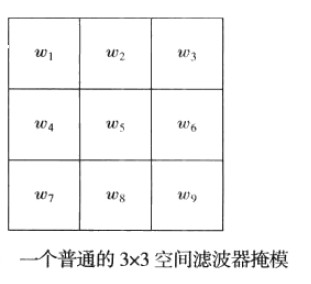2.1 - 孤立点的检测
点的检测以二阶导数为基础。这意味可以着使用拉普拉斯模板（详情见空间域滤波基础）
如果在某点该处模板的响应的绝对值超过了一个指定的阈值，那么我们	说在模板中心位置(x,y)处的该点已被检测到了。在输入图像中，这样的点被标注为1，而所有其他点则被标注为0，从而产生一副二值图像。
$g(x,y)=\begin{cases} 1& | R(x,y)| \geq T\\ 0 & \text{ 其他 } \end{cases}$
其中，g是输出图像，T是一个非负的阈值，R由上式给出。
2.2 - 线检测
复杂度更高的检测是线检测，对于线检测，可以预期二阶导数将导致更强的响应，并产生比一阶导数更细的线。这样对于线检测，我们也可以使用拉普拉斯模板，记住，二阶导数的双线效应必须做适当的处理。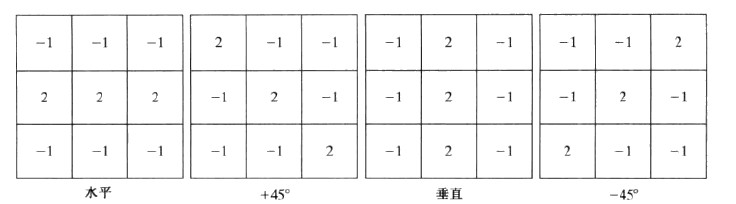2.4 边缘模型
边缘检测是基于灰度突变来分割图像最常用的方法。我们从介绍一些边缘建模的方法开始，然后讨论一些边缘检测的方法。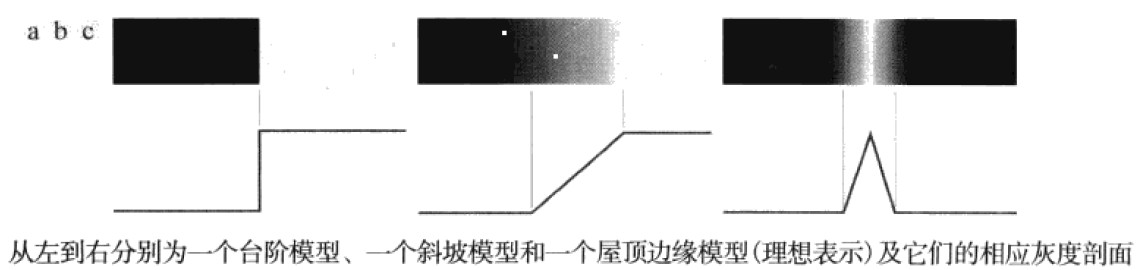实际中，数字图像都存在被模糊且带有噪声的边缘，模糊程度主要取决于聚焦机理中的兼职，而噪声水平主要取决于成像系统的电子元件。在这种情况下，边缘被建模为一个更接近灰度斜坡的剖面。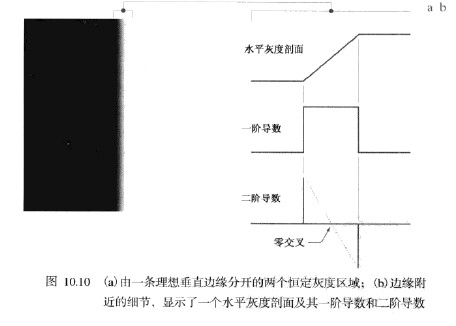并且我们可以得出结论：一阶导数的幅度可用于检测图像中的某个点是否存在一个边缘，二阶导数可以用于确定一个边缘像素位于该边缘的暗的一侧还是亮的一侧。
那么这是理想情况下的图片边缘，如果图片有噪声的话，其边缘函数则为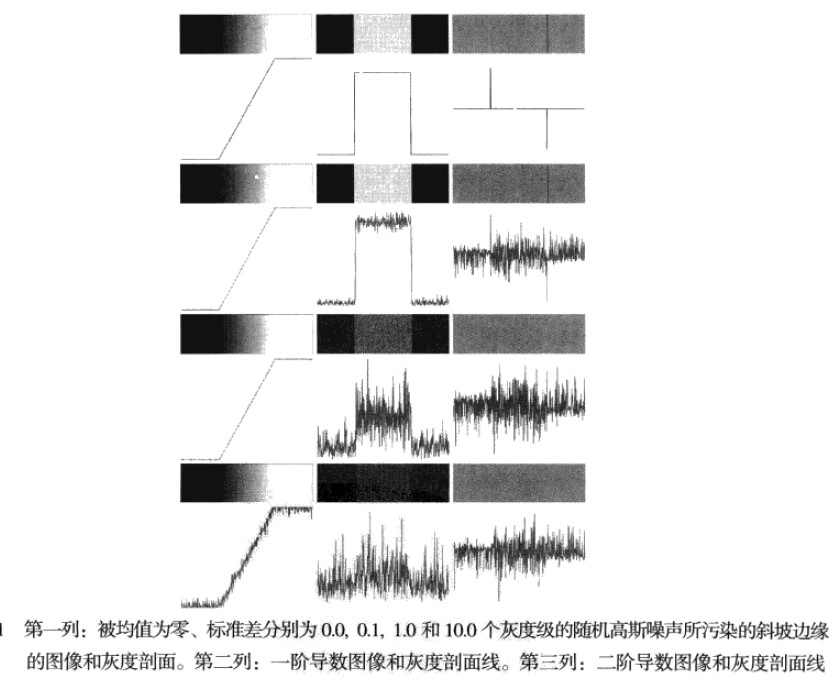微弱的可见噪声对检测边缘所用的两个关键导数的严重影响的这一事实，是我们应记住的一个重要问题。特别地，在类似于我们刚刚讨论的水平的噪声很可能存在的应用中，使用导数之前的图像平滑处理是应该认真考虑的问题。
因此边缘检测的三个基本步骤

为降噪对图像进行平滑处理
边缘点的检测
边缘定位

2.4.1 - 基本的边缘检测算子
Roberts算子
Roberts算子以求对角像素之差为基础，该算子用于识别对角线方向的边缘：
$g_x=(z_9-z_5)和g_y=(z_8-z_6)$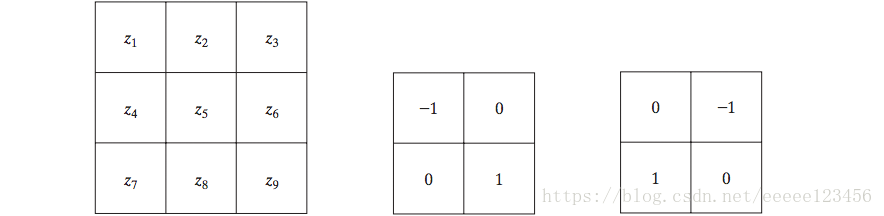Prewitt算子
Prewitt算子使用以$z_5$为中心的3x3领域对$g_x$和$g_y$的近似如下式所示
$g_x=(z_7+z_8+z_9)-(z_1+z_2+z_3)$
$g_y=(z_3+z_6+z_9)-(z_1+z_4+z_7)$
模板如下图：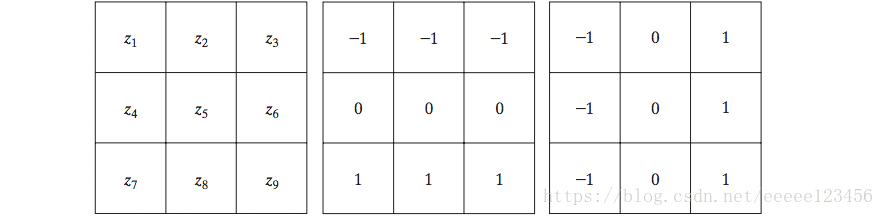Sobel算子
Sobel算子使用以$z_5$为中心的3x3领域对$g_x$和$g_y$的近似如下式所示：
$g_x=(z_7+2z_8+z_9)-(z_1+2z_2+z_3)$
$g_y=(z_3+2z_6+z_9)-(z_1+2_z4+z_7)$
Sobel模板能较好地抑制（平滑）噪声地特性使得它更为可取，因为在处理导数时噪声抑制是一个重要地问题。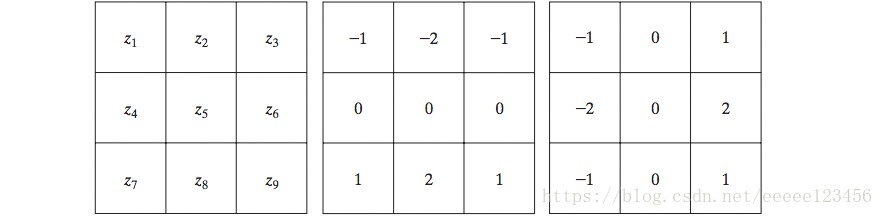检测对角边缘的Prewitt和Sobel模板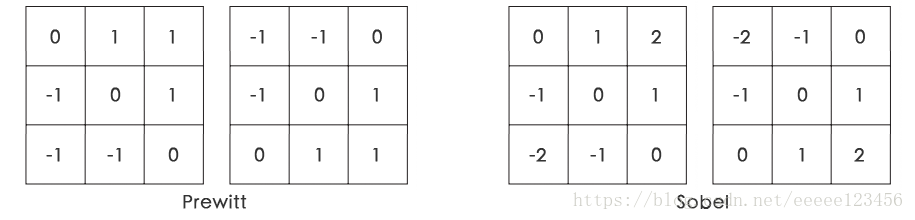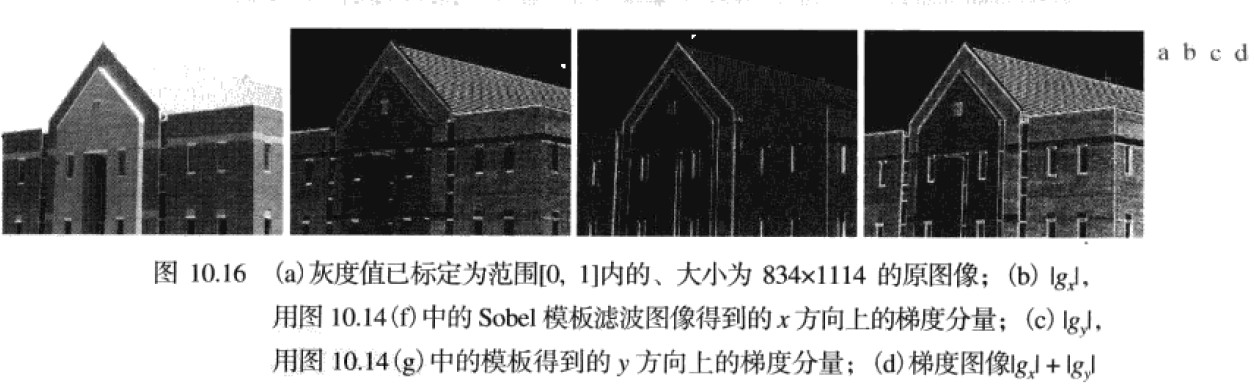3 - 成熟先进的边缘检测技术
3.1 - Marr-Hildreth边缘检测器
① 概念背景：
最早的成功地尝试将更高级的分析结合到边缘检测处理之一应归功与Marr和Hildreth。
Marr和Hildreth证明了：

灰度边缘与图像尺寸无关，因此他们的检测要求使用不同尺寸的算子；
灰度的突然变化会在一阶导数中引起波峰或波谷，或在二阶导数中等效地引起零交叉

这些概念建议，用于边缘检测的算子应有两个显著的特点。

第一个和最重要的特点是它应该是一个能计算图像中每一点处的一阶导数或二阶导数的数字近似的微分算子。
第二个是它应能被“调整”以便在任何期望的尺寸上起作用
因此，大的算子也可以用于检测模糊边缘，小的算子也可以用于检测锐度集中的精细细节。

② 高斯拉普拉斯(LoG)：
Marr和Hildreth证明了：满足这些最令人满意的算子是滤波器$\triangledown^2G$,$\triangledown^2$是拉普拉斯算子（$\partial^2/\partial x^2+\partial^2/\partial y^2$）,而G是标准差为$\sigma$的二维高斯函数
$G(x,y)=e^{-\frac{x^2+y^2}{2\sigma^2}}$
为求$\triangledown^2G$的表达式，我们执行如下微分：
$\triangledown^2G(x,y)=\frac{\partial^2G(x,y)}{\partial x^2}+\frac{\partial^2G(x,y)}{\partial y^2}=\frac{\partial}{\partial x}[\frac{-x}{\sigma^2}e^{-\frac{x^2+y^2}{2\sigma^2}}]+\frac{\partial}{\partial y}[\frac{-y}{\sigma^2}e^{-\frac{x^2+y^2}{2\sigma^2}}]$
整理各项后给出如下最终表达式：
$\triangledown^2G(x,y)=[\frac{x^2+y^2-2\sigma^2}{\sigma^4}]e^{-\frac{x^2+y^2}{2\sigma^2}}$
该表达式成为高斯拉普拉斯（LoG）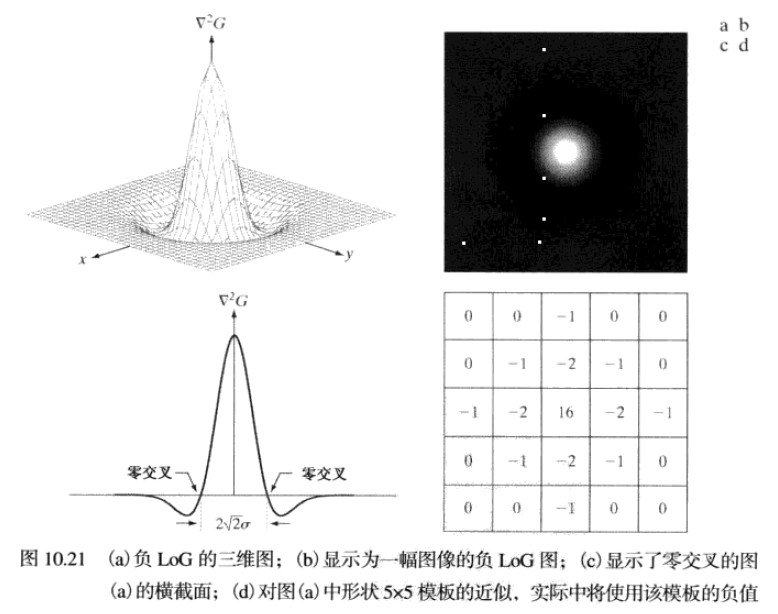③ Marr-Hildreth算法
Marr-Hildreth算法由LOG滤波器与一副输入图像f(x,y)卷积组成，即
$g(x,y)=[\triangledown^2G(x,y)]\bigstar f(x,y)$
然后找寻g(x,y)的零交叉来确定f(x,y)中边缘的位置。因为这些都是线性操作，故可以写为
KaTeX parse error: Expected 'EOF', got '\bigstarf' at position 30: …ledown^2[G(x,y)\̲b̲i̲g̲s̲t̲a̲r̲f̲(x,y)]
它指出我们可以先使用一个高斯滤波器来平滑图像，然后计算该结果的拉普拉斯。
Marr-Hildreth算法小结

用一个G(x,y)取样得到的nxn的高斯高斯低通滤波器对输入图像滤波。
计算由第一步得到的图像的拉普拉斯
找到步骤2所有图像的零交叉

零交叉是Marr-Hildreth边缘检测方法的关键特征，实现简单，并且通常能给出好的结果。
import numpy as np
import matplotlib.pyplot as plt
import cv2

def edgesMarrHildreth(img, sigma):
"""
finds the edges using MarrHildreth edge detection method...
:param im : input image
:param sigma : sigma is the std-deviation and refers to the spread of gaussian
:return:
a binary edge image...
"""
size = int(2 * (np.ceil(3 * sigma)) + 1)

x, y = np.meshgrid(np.arange(-size / 2 + 1, size / 2 + 1), np.arange(-size / 2 + 1, size / 2 + 1))

normal = 1 / (2.0 * np.pi * sigma ** 2)

kernel = ((x ** 2 + y ** 2 - (2.0 * sigma ** 2)) / sigma ** 4) * np.exp(
-(x ** 2 + y ** 2) / (2.0 * sigma ** 2)) / normal  # LoG filter

kern_size = kernel.shape
log = np.zeros_like(img, dtype=float)

# applying filter
for i in range(img.shape - (kern_size - 1)):
for j in range(img.shape - (kern_size - 1)):
window = img[i:i + kern_size, j:j + kern_size] * kernel
log[i, j] = np.sum(window)

log = log.astype(np.int64, copy=False)

zero_crossing = np.zeros_like(log)

# computing zero crossing
for i in range(log.shape - (kern_size - 1)):
for j in range(log.shape - (kern_size - 1)):
if log[i][j] == 0:
if (log[i][j - 1] < 0 and log[i][j + 1] > 0) or (log[i][j - 1] < 0 and log[i][j + 1] < 0) or (
log[i - 1][j] < 0 and log[i + 1][j] > 0) or (log[i - 1][j] > 0 and log[i + 1][j] < 0):
zero_crossing[i][j] = 255
if log[i][j] < 0:
if (log[i][j - 1] > 0) or (log[i][j + 1] > 0) or (log[i - 1][j] > 0) or (log[i + 1][j] > 0):
zero_crossing[i][j] = 255

# plotting images
fig = plt.figure()
imgplot = plt.imshow(log, cmap='gray')
a.set_title('Laplacian of Gaussian')
imgplot = plt.imshow(zero_crossing, cmap='gray')
string = 'Zero Crossing sigma = '
string += (str(sigma))
a.set_title(string)
plt.show()

return zero_crossing

img = edgesMarrHildreth(img,4)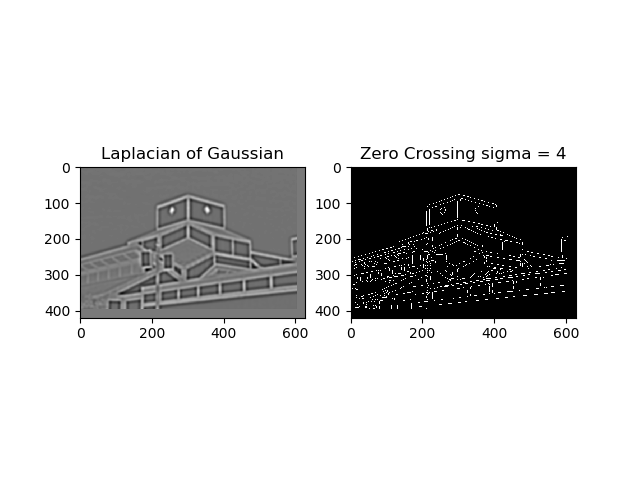（可以看到这个算法检测出了图像的边缘，但是有很多地方都是不连续的，那么之后我们会介绍如何检测边界和边缘连接）
3.2 - 坎尼边缘检测（Canny）
虽然其算法更为复杂，但是Canny边缘检测是迄今为止讨论过的边缘检测器中最为优秀的，Canny基于三个基本目标：

低错误率。所有边缘都应被找到，并且应该没有伪相应，也就是检测到的边缘必须尽可能是真是的边缘
边缘点应被很好的定位。已定位边缘必须尽可能接近真实边缘。也就是由检测器标记为边缘的点和真实边缘的中心之间的距离应该最小
单一的边缘点响应。对于真实的边缘点，检测器仅应返回一个点。也就是真是边缘周围的局部最大数应该是最小的。这意味着在仅存一个单一边缘点到额位置，检测器不应指出多个边缘像素。

Canny工作的本质是，从数学上表达前面的三个准则，并试图找到这些表达式的最佳解，通常这是很困难的，但是我们可以使用高斯近似得出最优解：首先使用一个环形二维高斯函数平滑图像，计算结果的梯度，然后使用梯度幅度和方向来估计每一点的边缘强度与方向
第一步
令f(x,y)表示输入图像，G(x,y)表示高斯函数：
$G(x,y)=e^{-\frac{x^2+y^2}{2\sigma^2}}$
我们用G和f卷积形成一幅平滑的图像$f_s(x,y)$：
$f_s(x,y)=G(x,y)\bigstar f(x,y)$
第二步
接下来计算结果的梯度幅度和方向：
$M(x,y)=\sqrt {g_x^2+g_y^2}$
$\alpha(x,y)=arctan[\frac{g_y}{g_x}]$
第三步
细化边缘，使用非最大抑制：

寻找最接近$\alpha(x,y)$的方向$d_k$
若$M(x,y)$的值至少小于沿$d_k$的两个零邻居之一，零$g_N(x,y)=0$(抑制)；否则令$g_N(x,y) = M(x,y)$,得到最大非抑制后的图像$g_N(x,y)$

第四步
最后操作时对$g_N(x,y)$进行阈值处理，以便减少伪边缘点，Canny算法使用两个阈值：低阈值$T_L$和高阈值$T_H$(Canny建议高低阈值比为2:1或3:1)
$g_{NH}=\left \{ g_N(x,y)\geq T_H\right\}$
$g_{NL}=\left \{ g_N(x,y)\geq T_L\right\}$
$g_{NH}= g_{NL}(x,y)-g_{NH}(x,y)$
$g_{NH}(x,y)$和$g_{NL}(x,y)$的非零像素可分别视为“强”和“弱”边缘像素。其中$g_{NH}(x,y)$为边缘点，$g_{NL}(x,y)$为候选点，对于候选点，如果与边缘点邻近，就标记为边缘点。
具体步骤如下：

在$g_{NH}(x,y)$中定位一下个未被访问的边缘像素p
$g_{NL}(x,y)$中与p是8邻接的像素标记为有效边缘像素
若$g_{NH}(x,y)$中的所有非零像素已被访问，则跳到步骤4，否走返回步骤1
将$g_{NL}(x,y)$中未标记为有效边缘像素的所有像素置零
在这一过程的末尾，将来自$g_{NL}(x,y)$的所有非零像素附近到$g_{NH}(x,y)$，用Canny算子形成最终的输出图像。

那么我们来总结一下Canny算法
Canny算法步骤

用一个高斯滤波器平滑输入图像
计算梯度幅度图像和角度图像
对梯度幅度图像应用非最大抑制
用双阈值处理和连接分析来检测并连接边缘（这相对Marr-Hildreth优化了边缘的连接使得检测的边缘更加完整）

OpenCV将这一算法封装成了一个函数
def Canny(image, threshold1, threshold2, edges=None, apertureSize=None, L2gradient=None): # real signature unknown; restored from __doc__
"""
Canny(image, threshold1, threshold2[, edges[, apertureSize[, L2gradient]]]) -> edges
.   @brief Finds edges in an image using the Canny algorithm @cite Canny86 .
.
.   The function finds edges in the input image and marks them in the output map edges using the
.   Canny algorithm. The smallest value between threshold1 and threshold2 is used for edge linking. The
.   largest value is used to find initial segments of strong edges. See
.   <http://en.wikipedia.org/wiki/Canny_edge_detector>
.
.   @param image 8-bit input image.
.   @param edges output edge map; single channels 8-bit image, which has the same size as image .
.   @param threshold1 first threshold for the hysteresis procedure.
.   @param threshold2 second threshold for the hysteresis procedure.
.   @param apertureSize aperture size for the Sobel operator.
.   @param L2gradient a flag, indicating whether a more accurate \f$L_2\f$ norm
.   \f$=\sqrt{(dI/dx)^2 + (dI/dy)^2}\f$ should be used to calculate the image gradient magnitude (
.   L2gradient=true ), or whether the default \f$L_1\f$ norm \f$=|dI/dx|+|dI/dy|\f$ is enough (

必要参数：

第一个参数是需要处理的原图像，该图像必须为单通道的灰度图；
第二个参数是阈值1；
第三个参数是阈值2。

函数返回一副二值图，其中包含检测出的边缘。
使用
Canny函数的使用很简单，只需指定最大和最小阈值即可。如下：
# coding=utf-8
import cv2
import numpy as np

cv2.imshow('img',img)
img = cv2.GaussianBlur(img, (3, 3), 0)
canny = cv2.Canny(img, 50, 150)

cv2.imshow('Canny', canny)
cv2.waitKey(0)
cv2.destroyAllWindows()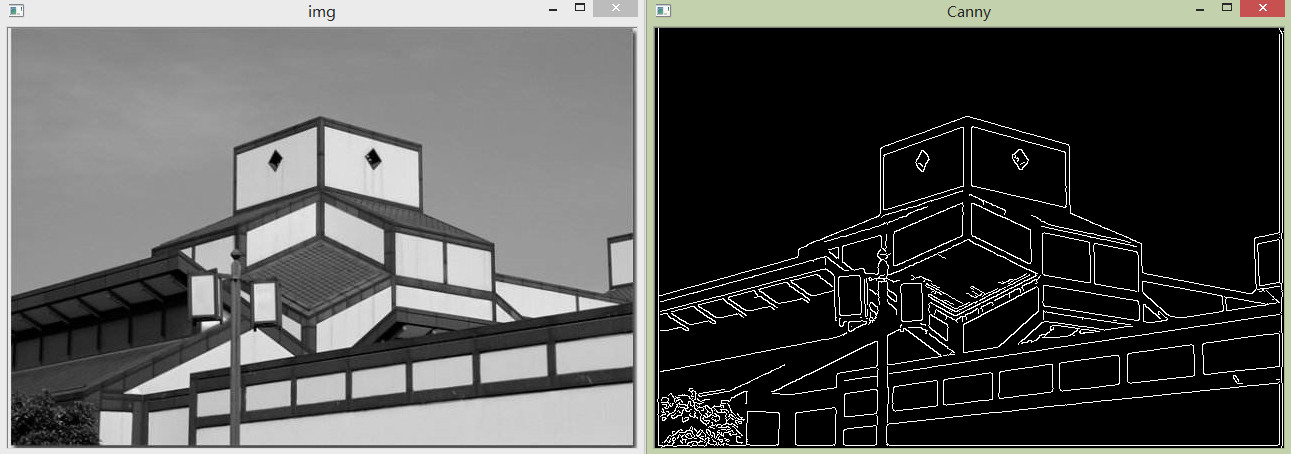可以看到，Canny算法明显提取边缘的效果要优于Marr-Hildreth算法，对边缘的连接也做的更好。


展开全文• 本文主要整理自笔者在一项图像处理任务中的直线检测（line detection）部分的笔记资料，采用了基于霍夫变换（Hough Transform）的直线检测算法。文中给出了直线检测常用的算法介绍，论文资料等，以及笔者的实验笔记...
前言

[图像处理] 实验笔记系列是以图像处理算法为主的文章专栏，以我在算法研究中的实验笔记资料为基础加以整理推出的。该系列内容涉及常见的图像处理算法理论以及常见的算法应用，每篇博客都会介绍相关的算法原理，代码实现和算法在实际应用中的技巧。
本文主要整理自笔者在一项图像处理任务中的直线检测（line detection）部分的笔记资料，采用了基于霍夫变换（Hough Transform）的直线检测算法。文中给出了直线检测常用的算法介绍，论文资料等，以及笔者的实验笔记和实验结果。

文章小节安排如下：
1）直线检测相关算法
2）霍夫直线检测的基本原理
3）霍夫直线检测的OpenCV实现
4）直线检测的应用

一、直线检测相关算法

1.1 霍夫变换（Hough Transform）

霍夫变换（Hough Transform）换于1962年由Paul Hough 首次提出，后于1972年由Richard Duda和Peter Hart推广使用，是图像处理中从图像中检测几何形状的基本方法之一。经典霍夫变换用来检测图像中的直线，后来霍夫变换经过扩展可以进行任意形状物体的识别，例如圆和椭圆。

霍夫变换运用两个坐标空间之间的变换将在一个空间中具有相同形状的曲线或直线映射到另一个坐标空间的一个点上形成峰值，从而把检测任意形状的问题转化为统计峰值问题。

参考论文：
 P.V.C. Hough,Machine Analysis of Bubble Chamber Pictures, Proc. Int. Conf. High Energy Accelerators and Instrumentation, 1959.
 Duda, R. O. and P. E. Hart, “Use of the Hough Transformation to Detect Lines and Curves in Pictures,”Comm. ACM, Vol. 15, pp. 11–15 (January, 1972).

1.2 霍夫直线检测（Hough Line Detection）

Hough直线检测的基本原理在于利用点与线的对偶性，在我们的直线检测任务中，即图像空间中的直线与参数空间中的点是一一对应的，参数空间中的直线与图像空间中的点也是一一对应的。这意味着我们可以得出两个非常有用的结论：
1）图像空间中的每条直线在参数空间中都对应着单独一个点来表示；
2）图像空间中的直线上任何一部分线段在参数空间对应的是同一个点。

因此Hough直线检测算法就是把在图像空间中的直线检测问题转换到参数空间中对点的检测问题，通过在参数空间里寻找峰值来完成直线检测任务。

1.3 LSD

-待续-

二、霍夫直线检测的基本原理

2.1 关于对偶性

首先，我们通过实例来解释一下对偶性的意义。
1）图像空间中的点与参数空间中的直线一一对应
在图像空间x-y中一条直线在直角坐标系下可以表示为：其中k和b是参数，对应表示斜率和截距。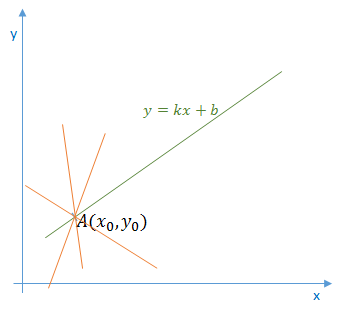过某一点A(x0, y0)的所有直线的参数均满足方程y0=k*x0+b，即点A(x0, y0)确定了一族直线。
如果我们将方程改写为：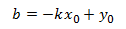那么该方程在参数空间k-b中就对应了一条直线：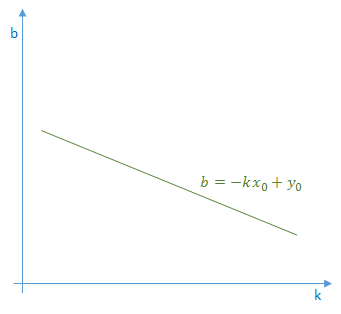也就是说，图像空间x-y中的点(x0,y0)对应了参数空间k-b中的直线b=-k*x0+y0。因此可以得到结论，图像空间中的点与参数空间中的直线一一对应。

2）图像空间中的直线与参数空间中的点一一对应
我们在直线y=k*x+b上再增加一个点B(x1, y1)，如下图所示：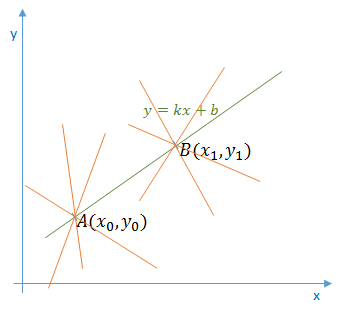那么点B(x1, y1)在参数空间同样对应了一条直线：可以看到，图像空间x-y中的点A和点B在参数空间k-b中对应的直线相交于一点，这也就是说AB所确定的直线，在参数空间中对应着唯一一个点，这个点的坐标值（k0, b0）也就是直线AB的参数。

以上就是在直线检测任务中关于对偶性的直观解释。这个性质也为我们解决直线检测任务提供了方法，也就是把图像空间中的直线对应到参数空间中的点，最后通过统计特性来解决问题。假如图像空间中有两条直线，那么最终在参数空间中就会对应到两个峰值点，依此类推。

2.2 参数空间的选择

上述为了方便讲解对偶性和霍夫变换的基本原理，我们的参数空间也选择了笛卡尔直角坐标系。但在实际应用中，参数空间是不能选择直角坐标系的，因为原始图像直角坐标空间中的特殊直线x=c（垂直x轴，直线的斜率为无穷大）是没办法在基于直角坐标系的参数空间中表示的。

所以在实际应用中，参数空间采用极坐标系ρ-θ，图示如下：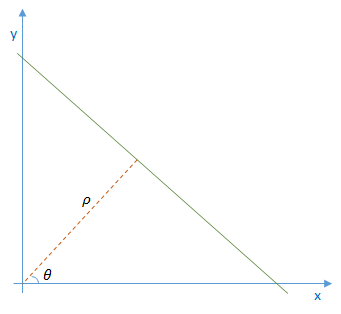直线的表达式为：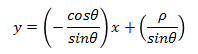化简便可得到：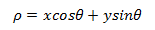对于直线上的点(x0, y0)，可以将通过该点的直线族定义为：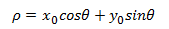这就回到我们刚才的结论，参数空间的每个点(ρ,θ)都对应了图像空间的一条直线，或者说图像空间的一个点在参数空间中就对应为一条曲线。参数空间采用极坐标系，这样就可以在参数空间表示原始空间中的所有直线了。
注意，此时图像空间（直角坐标系x-y）上的一个点对应到参数空间（极坐标系ρ-θ）上是一条曲线，确切的说是一条正弦曲线。2.3 利用霍夫变换检测直线

如前所述，霍夫直线检测就是把图像空间中的直线变换到参数空间中的点，通过统计特性来解决检测问题。具体来说，如果一幅图像中的像素构成一条直线，那么这些像素坐标值（x, y）在参数空间对应的曲线一定相交于一个点，所以我们只需要将图像中的所有像素点（坐标值）变换成参数空间的曲线，并在参数空间检测曲线交点就可以确定直线了。

在理论上，一个点对应无数条直线或者说任意方向的直线，但在实际应用中，我们必须限定直线的数量（即有限数量的方向）才能够进行计算。

因此，我们将直线的方向θ离散化为有限个等间距的离散值，参数ρ也就对应离散化为有限个值，于是参数空间不再是连续的，而是被离散量化为一个个等大小网格单元。将图像空间（直角坐标系）中每个像素点坐标值变换到参数空间（极坐标系）后，所得值会落在某个网格内，使该网格单元的累加计数器加1。当图像空间中所有的像素都经过霍夫变换后，对网格单元进行检查，累加计数值最大的网格，其坐标值（ρ0, θ0）就对应图像空间中所求的直线。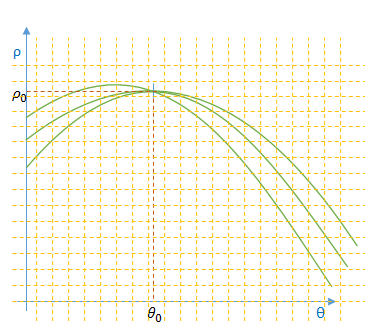以上就是霍夫直线检测算法要做的，它检测图像中每个像素点在参数空间对应曲线之间的交点，如果交于一点的曲线的数量超过了阈值，那就可以认为这个交点(ρ,θ)在图像空间中对应一条直线。

2.4 霍夫直线检测的优缺点

优点：
Hough直线检测的优点是抗干扰能力强，对图像中直线的殘缺部分、噪声以及其它共存的非直线结构不敏感。
缺点：
Hough变换算法的特点导致其时间复杂度和空间复杂度都很高，并且在检测过程中只能确定直线方向，丢失了线段的长度信息。

三、霍夫直线检测的OpenCV实现

OpenCV支持三种霍夫直线检测算法：
1）Standard Hough Transform(SHT，标准霍夫变换)
2）Multiscale Hough Transform(MSHT，多尺度霍夫变换)
3）Progressive Probability Houth Transform(PPHT，渐进概率式霍夫变换)

3.1 霍夫直线检测函数定义

在OpenCV2.1之前的版本，霍夫直线检测函数如下：

函数原型：

CVAPI(CvSeq*)  cvHoughLines2( CvArr* image, void* line_storage, int method,
double rho, double theta, int threshold,
double param1 CV_DEFAULT(0), double param2 CV_DEFAULT(0),
double min_theta CV_DEFAULT(0), double max_theta CV_DEFAULT(CV_PI));

函数说明：
cvHoughLines2老版OpenCV的霍夫直线检测函数，通过method参数可以支持三种霍夫直线检测算法，分别是CV_HOUGH_STANDARD、CV_HOUGH_PROBABILISTIC =1、CV_HOUGH_MULTI_SCALE。

在OpenCV新版本下，霍夫直线检测算法定义了两个函数：HoughLines、HoughLinesP
1）HoughLines：标准霍夫变换、多尺度霍夫变换
函数原型：

CV_EXPORTS_W void HoughLines( InputArray image, OutputArray lines,
double rho, double theta, int threshold,
double srn = 0, double stn = 0,
double min_theta = 0, double max_theta = CV_PI );

参数说明：
InputArray image：输入图像，必须是8位单通道图像。
OutputArray lines：检测到的线条参数集合。
double rho：以像素为单位的距离步长。
double theta：以弧度为单位的角度步长。
int threshold：累加计数值的阈值参数，当参数空间某个交点的累加计数的值超过该阈值，则认为该交点对应了图像空间的一条直线。
double srn：默认值为0，用于在多尺度霍夫变换中作为参数rho的除数，rho=rho/srn。
double stn：默认值为0，用于在多尺度霍夫变换中作为参数theta的除数，theta=theta/stn。

函数说明：
HoughLines函数输出检测到直线的矢量表示集合，每一条直线由具有两个元素的矢量（ρ, θ）表示，其中ρ表示直线距离原点（0, 0）的长度，θ表示直线的角度（以弧度为单位）。
HoughLines函数无法输出图像空间中线段的长度，这也是霍夫变换本身的弱点。

备注说明：
如果srn和stn同时为0，就表示HoughLines函数执行标准霍夫变换，否则就是执行多尺度霍夫变换。

2）HoughLinesP：渐进概率式霍夫变换
函数原型：

CV_EXPORTS_W void HoughLinesP( InputArray image, OutputArray lines,
double rho, double theta, int threshold,
double minLineLength = 0, double maxLineGap = 0 );

参数说明：
InputArray image：输入图像，必须是8位单通道图像。
OutputArray lines：检测到的线条参数集合。
double rho：直线搜索时的距离步长，以像素为单位。
double theta：直线搜索时的角度步长，以弧度为单位。
int threshold：累加计数值的阈值参数，当参数空间某个交点的累加计数的值超过该阈值，则认为该交点对应了图像空间的一条直线。
double minLineLength：默认值为0，表示最小线段长度阈值（像素）。
double maxLineGap：默认值为0，表示直线断裂的最大间隔距离阈值。即如果有两条线段是在一条直线上，但它们之间有间隙，那么如果这个间隔距离大于该值，则被认为是一条线段，否则认为是两条线段。

函数说明：
HoughLinesP函数输出检测到直线的矢量表示集合，每一条直线由具有四个元素的矢量（x1, y1, x2, y2）表示，其中(x1, y1)表示线段的起点，(x2, y2)表示线段的终点。
HoughLinesP函数可以检测出图像空间中线段的长度。

3.2 霍夫直线检测函数使用

霍夫直线变换是一种用来在图像空间寻找直线的方法，输入图像要求是二值图像，同时为了提高检测直线的效率和准确率，在使用霍夫线变换之前，最好对图像进行边缘检测生成边缘二值图像，这样的检测效果是最好的。

1）HoughLines函数
代码：

std::string img_path;
cv::Mat mat_color;
cv::Mat mat_gray;
cv::Mat mat_binary;
cv::Mat mat_canny;
cv::Mat mat_board;

img_path = "line.png";
mat_board = cv::Mat(mat_color.size(), mat_color.type(), Scalar::all(255));

// binary
cv::threshold(mat_gray, mat_binary, 0.0, 255.0, cv::THRESH_OTSU);
// invert color
cv::bitwise_not(mat_binary, mat_binary);

// detect edge
Canny(mat_binary, mat_canny, 50, 200, 3);

// detect line
vector<Vec2f> lines;
HoughLines(mat_canny, lines, 1, CV_PI / 180, 150, 0, 0);

// draw line
cout << "line number: " << lines.size() << endl;
for (size_t i = 0; i < lines.size(); i++)
{
Vec2f linex = lines[i];
cout << "radius: " << linex << ", radian: "<< linex << ", angle: " << 180 / CV_PI * linex << endl;
float rho = lines[i], theta = lines[i];
Point pt1, pt2;
double a = cos(theta), b = sin(theta);
double x0 = a * rho, y0 = b * rho;
pt1.x = cvRound(x0 + 1000 * (-b));
pt1.y = cvRound(y0 + 1000 * (a));
pt2.x = cvRound(x0 - 1000 * (-b));
pt2.y = cvRound(y0 - 1000 * (a));
line(mat_board, pt1, pt2, Scalar(255, 0, 0), 1);
}
cv::imshow("gray", mat_gray);
cv::imshow("binary", mat_binary);
cv::imshow("canny", mat_canny);
cv::imshow("color", mat_board);
cv::waitKey();

原图：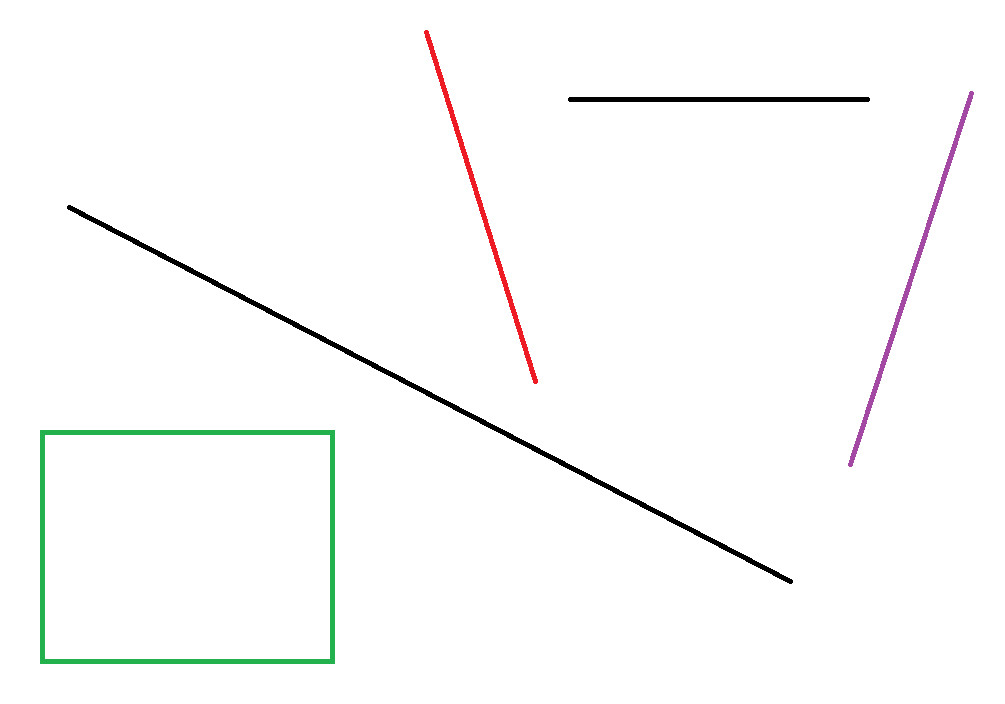二值图：边缘图：检测效果：2）HoughLinesP函数
代码：

std::string img_path;
cv::Mat mat_color;
cv::Mat mat_gray;
cv::Mat mat_binary;
cv::Mat mat_canny;
cv::Mat mat_board;

img_path = "line.png";
mat_board = cv::Mat(mat_color.size(), mat_color.type(), Scalar::all(255));

// binary
cv::threshold(mat_gray, mat_binary, 0.0, 255.0, cv::THRESH_OTSU);
// invert color
cv::bitwise_not(mat_binary, mat_binary);

// detect edge
Canny(mat_binary, mat_canny, 50, 200, 3);

// detect line
vector<Vec4i> lines;
HoughLinesP(mat_canny, lines, 1, CV_PI / 180, 150, 50, 50);

// draw line
cout << "line number: " << lines.size() << endl;
for (size_t i = 0; i < lines.size(); i++)
{
Vec4i linex = lines[i];
line(mat_board, Point(linex, linex), Point(linex, linex), Scalar(255, 0, 0), 1);
}

cv::imshow("gray",   mat_gray);
cv::imshow("binary", mat_binary);
cv::imshow("canny", mat_canny);
cv::imshow("color", mat_board);
cv::waitKey();

检测效果：四、直线检测的应用

4.1 直线检测的实际应用

直线检测是机器视觉和模式识别中最重要的任务之一，对图像理解/分析等有重要的意义。在实际应用中，直线检测可用于机器人定位中的网格识别，板材的裂纹检测，表单票据的格式识别，零件纹路的检测，自动驾驶中的车道检测等等，可以看出，在工业领域中，直线检测以及各种图像处理技术应用是非常丰富的。

4.2 直线检测在图像矫正方面的应用

笔者最近在一个OCR项目也使用了Hough Line Detection算法。在该项目中，待识别文本图像的内容是倾斜的（即文字是倾斜的），我们采用的测略就是通过直线检测确定图像内容的倾斜程度并进行旋转纠正，这样得到的无倾斜图像更有利于OCR任务。
原图：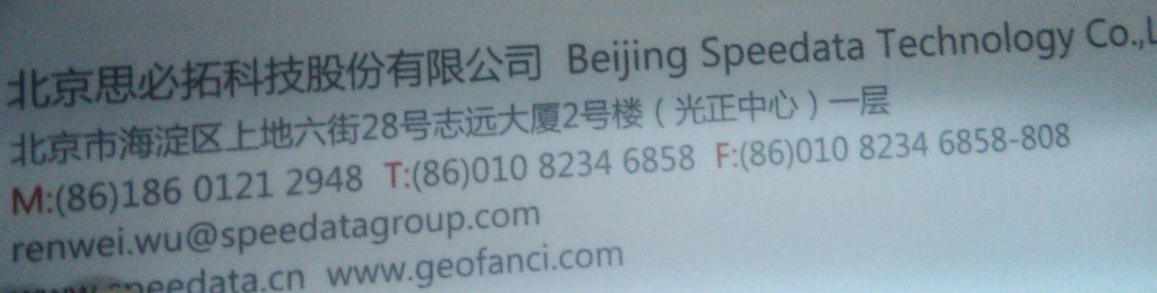矫正效果：五、参考资料

参考论文与书目：
 Duda, R. O. and P. E. Hart, “Use of the Hough Transformation to Detect Lines and Curves in Pictures,”Comm. ACM, Vol. 15, pp. 11–15 (January, 1972).
 顾思妍. 机器视觉的直线检测技术及应用研究[D].广东工业大学,2011.
 数字图像处理[M]. 电子工业出版社 , (美)RafaelC.Gonzalez,(美)RichardE.Woods,(美)StevenL.Eddins著, 2005

参考博客：
Hough变换-理解篇
http://blog.csdn.net/abcjennifer/article/details/7448513
Hough transform(霍夫变换)
http://www.cnblogs.com/AndyJee/p/3805594.html
霍夫变换概述和标准霍夫变换
http://www.jianshu.com/p/55eabb42c6c2`
展开全文图像处理 算法 霍夫变换
• 1.Euclidean Distance Euclidean Distance(欧氏距离)是一种常用的度量方式，是点和点之间...Manhattan Distance(曼哈顿距离)也称为街区距离，表示对点与点之间在不同维度上的绝对距离的叠加，它的定义如下： 3.C
• 一、边缘检测算子类别 常见边缘检测算子：Roberts 、Sobel 、Prewitt、Laplacian、Log/Marr、Canny、Kirsch、Nevitia二、一阶微分算子：Roberts 、Sobel 、Prewitt Robert算子是第一个边缘检测算子，提出者...边缘检测算子
•  1、图像锐化和边缘检测的基本概念，微分梯度已经差分的定义  2、锐化和边缘检测的像素处理方式（3种）  3、单方向一阶微分锐化，包括：  水平方向  垂直方向  Kirsch算子  4、无方向微分锐化，包括： ...
• 边界聚类椭圆检测算法经典椭圆检测方法投票（聚类）方法随机hough变换椭圆检测算法最优化方法基于弧段的方法边界聚类算法流程预处理边界像素连接线段列提取线段列旋转方向统一凹点和角点检测圆弧聚类再配对直接最小...
• 边缘是图像中像素值发生剧烈变化而不连续的结果，它存在于目标与背景、目标与目标、区域与区域之间。边缘检测是图像基于边界分割的第一步。由图像灰度的特点，可将边缘类型分为阶梯状边缘（处于图像两个具有不同灰度...
• 该算子包含两组3*3的矩阵，分别为图像横向及纵向，将之与图像作平面卷积，即可分别得出横向及纵向的亮度差分近似值。如果以A代表原始图像，Gx及Gy分别代表经横向及纵向边缘检测的图像灰度值，其公式如下： 具体...图像处理
• 动态轮廓是图像分割的一个热点，从早期的snake，就有很多的优化版，测地线动态轮廓（GAC）就是其中之一。总体来说，其摒弃了snake对参数的依赖，并加入了水平集，使得轮廓曲线更贴近目标物的拓扑结构。 经典的动态...分割
• 演示应用Hough变换检测图像中得圆,得到检测目标,详解讲述了这一算法过程 并且展示了算法运行的效果. 讨论了hough变换检测的先决条件.
• 数字图像处理数字图像处理 一学习内容总结 第一章 绪论 1 什么是数字图像处理 2 使用数字图像处理领域的实例 3 数字图像处理的基本步骤 4 图像处理系统的组成 第二章 数字图像处理基础 1 视觉感知要素 2 光和电磁...数字图像处理
• 图像处理与识别学习小结 数字图像处理是对图像进行分析、加工、和处理，使其满足视觉、心理以及其他要求的技术。图像处理是信号处理在图像域上的一个应用。目前大多数的图像是以数字形式存储，因而图像处理很多情况...图像处理 processing 算法 image
•  在ocr（数字图像文本识别）过程中，由于图像的不可控性，总会存在一定角度的倾斜。倾斜角度要满足一定范围：   θ≤d/L （θ即倾斜角度，d为文本行距，L为文本行长）  若超出这个范围，则可能将下（上）...图像处理
• void CTrafficsampleDlg::OnOK() //读入视频并检测车辆 ...//此处添加图像处理的代码 //CDialog::OnOK(); IplImage *frame = NULL; //定义帧,每帧就是一张图 IplImage *B_part = NULL, *G_part =图像处理 null validation
• 1、象素间各种距离的定义及计算 我们知道，构成一幅数字图像最基本的元素是一个一个的像素点，也就是像素。理解像素间的一些基本关系是我们以后进行图形图形处理的基础和关键。如相邻像素（像素的邻域），像素的...
...